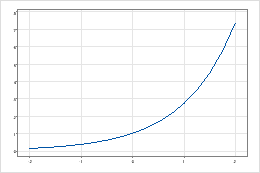# Exponential function

To use this function, choose Calc > Calculator.

Calculates the value ex, where e is the base of the natural log equal to approximately 2.71828 and x is the value that you enter. For example, the exponential of 5 is e5, which equals about 148.413. Usually, the function y = ex is called the natural exponential function.

###### Note

Some calculators define the Exponential function as the Antilog function in base e. In Minitab, the Antilog function is the inverse function for log base 10.

## Syntax

EXP(number)

In number, specify the value or column of values.

## Example

Calculator expression Result
EXP(2) 7.38905609893

## Uses

The Exponential function is often used to model amounts that increase or decrease by a constant exponential factor, such as compound interest, radioactive decay, or population growth.y = exAs x increases, y increases exponentially.
By using this site you agree to the use of cookies for analytics and personalized content.  Read our policy